# How to find the median

## How to find the median for odd, even and classified data sampling is explained in a simple way.

### Definition of median or central value

The median divides the frequency distribution as accurately into two halves as possible. It's other meaning central value got named after its position in the center of a distribution, one half of the distribution is smaller than the median and the other half bigger than it. An advantage of the median is its resistance against extreme high or low values which may disrupt the statistical outcome which the arithmetical mean may suffer from. Its disadvantage is that it is more difficult to calculate and that you have to put more work into it than the other way round. I will show you how to find the median by squirrels which collect hazelnuts.

##### eBay is a good source to find booksDSM-5 Diagnostic and Statistical Manual of Mental Disorders Ed...Time left: 3 weeks, 6 daysStatistics for Dummies? by Deborah RumseyTime left: 2 weeks, 6 daysStatistics for People Who (Think They) Hate Statistics 6th Edi...Time left: 2 weeks, 4 days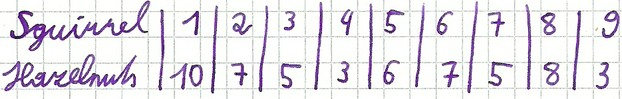Median even distribution

### How to find the median for an odd distribution

I start with this example, because it is the simplest possible setting. I have discovered nine squirrels in the woods and each of them had collected a different number of hazelnuts in their pockets. The first step is that the squirrels must be sorted in ascending order after the number of collected hazelnuts in their pockets. Squirrels 4 and 9 only have 3 hazelnuts in their pockets which is the lowest amount of collected hazelnuts and therefore they are assigned index x1 and x2. Five hazelnuts each have collected squirrel 3 and 7. Five is the second lowest number of collected hazelnuts and so the squirrels are awarded with index x3 and x4. Squirrel 5 has collected 6 hazelnuts which was not to bad and the payout is index x5 for the 6 hazelnuts. The next step forward concerning the hazelnuts are 7 collected hazelnuts by squirrel 2 and squirrel 6 which get marked with index x5, and x6. Eight hazelnuts for squirrel 8 – a diligent little fellow!. The last on the list is squirrel 1 which has 10 hazelnuts in its pocket which is indexed x9. All in all we have 9 squirrels on our list and so our sample volume is n=9 and our indexes are as follows x1=3, x2=3, x3=5, x4=5, x5=6, x6=7, x7=7, x8=8, x9=10. Now we only need to insert the data into the formula and voilà! The cake is baked. (n+1)/2=(9+1)/2=5 amd therefore the median is at index number 5 x5=6 hazelnuts. Exactly half of our squirrel collected less than 6 hazelnuts and the other half more than 6 hazelnuts.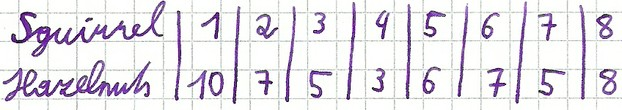Median even distribution

### How to find the median in an even distribution

On another day in the woods I discovered only eight squirrels. Eight squirrels mean our sample is n=8 and therefore the formula for an even distribution needs to be used. The first step is sort the squirrels again in ascending order by the number of hazelnuts in their pockets. x1 = 3, x2 = 5, x3 = 5, x4 = 6, x5 = 7, x6 = 7 = 8 x7, x8 = 10. The second step is to insert the data into the formula n/2=8/2=4 which is x4 and (n+2)/2=(8+2)/2=5 so x5. These indexes inserted into the formula 1/2*(x4+x5)=1/2*(6+7)=6,5 the result is 6,5 hazelnuts. So the median x~=6,5 is six and half hazelnut.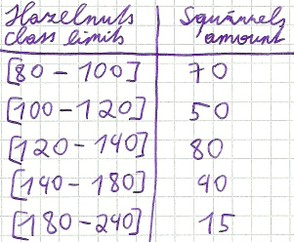Median classified distributionTeacher Made Math Center Learning Resource Game Shapes Match-UpTime left: 1 monthCurrent bid: \$6.50  Place bidDINO MATH TRACKS BOARD GAME, NEW SEALEDTime left: 1 day, 2 hoursCurrent bid: \$3.25  Place bid

### How to find the median for classified data

For binned data, the median or central value is somewhat more difficult to calculate, but if you define the formula as a story then it is a piece of cake. The step on our road to the median is to find the class in which the median is hiding itself. So all squirrels are added up and divided by two. N/2=(70+50+80+40+15)/2=127,5. That means the median is somwhere in the third class. Half the work is done! Now it is the same as always – sticking to the formula. All squirrels are added together and divided by 2. n / 2 = (70 +50 +80 +40 +15) / 2 = 127.5 Thus, the median in the third class occur somewhere within the third class, the sample is divided into two halves. Now it goes blow-by-blow on our way to calculate the median. Lower class limit ui = 120, class range bi is higher class limit minus lower class limit bi=140-120=20, relative frequency of the median-class is number of squirrels in the median-class divided by the total amount of squirrels hi=80/255=~0,31, relative cumulated frequency of all the classes before the median-class is number of squirrels before the median-class divided by the total amount of squirrels Hi=(70+50)/255=~0,47. Now at last we almost got our cake baked to get our piece of cake called median of classified data. The data inserted into the formula results in x~=ui+(0,5-Hi)/hi*bi=120+(0,5-0,47)/0,33*20=121,82. The median is x~=121,82 hazelnuts.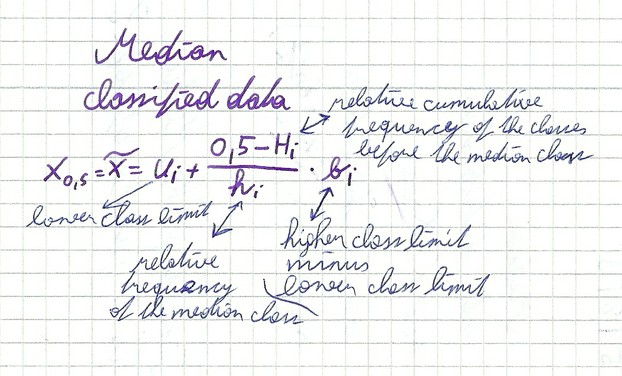Median formula for classified distribution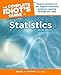The Complete Idiot's Guide to Statistics, 2nd EditionNot a numbers person? No problem! This new edition is aimed at high school and college students who need to take statistics to fulfill a degree requirement and follows a ...Alpha  / Only \$19.95
5

Only logged-in users are allowed to comment. Login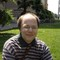;) I also got a handful of hazelnuts from my colleague at university. I like them too.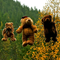sheilamarie on 12/18/2011

Busy little squirrels! They really did eat my hazelnuts this year, at least the ones the bears didn't already gobble. Now that you've emptied the pockets of the squirrels, are you brave enough to tackle the median number of hazelnuts the bears have taken from my tree? You could even do that median over a number of years, as you only need one hungry bear to strip the tree in the autumn.

#### You might also like

The Engineering Mastery of the Wright Brothers

Most people recognize that the Wright brothers mastered powered flight, but f...

The Magic and Myth of British Fungi

Mushrooms and toadstools grow all over the world, and whilst many are edible ...

Disclosure: This page generates income for authors based on affiliate relationships with our partners, including Amazon, Google and others.